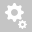##Recently viewed ticketsKnowledge base » FAQ for midas Civil » [ANALYSIS]     What is the basis of P-∆ analysis in midas Civil? secondary moment from P-∆ analysis matches with classical method calculation using actual stress rather failure stress. why?

# [ANALYSIS]     What is the basis of P-∆ analysis in midas Civil? secondary moment from P-∆ analysis matches with classical method calculation using actual stress rather failure stress. why?

P-∆ analysis in midas Civil is a real-time displacement analysis,
where- in as per the actual load application deflection is calculated and the stiffness matrix is modified accordingly.

The P-Delta analysis option in midas Civil is a type of Geometric nonlinearity,
which accounts for secondary structural behavior when axial and transverse loads are simultaneously applied to beam or wall elements.

The flow chart for P-Δ analysis in midas Civil is given belowLinear static analysis is performed first for a given loading condition and then a new geometric stiffness matrix is
formulated based on the member forces or stresses obtained from the first analysis.
The geometric stiffness matrix is thus repeatedly modified and used to perform subsequent static analyses
until the given convergence conditions are satisfied.

Virtually all design codes such as ACI 318 and AISC-LRFD specify that the P-Delta effect be included
in structural analyses to account for more realistic member forces.

The classical nominal curvature method is used for the calculation of curvature (1/r) using design strain,
for calculating secondary moments theoretically considering the design yield

strain and factoring it by load correction factors. The additional moment obtained in Midas are directly based on the actual
deflected shape of the member due to the applied loading. Thus comparing classical method results
calculated using actual stresses will yield results comparable with P-Δ analysis results.
Creation date: 6/22/2016 6:43 PM ()      Updated: 9/5/2017 9:45 AM ()